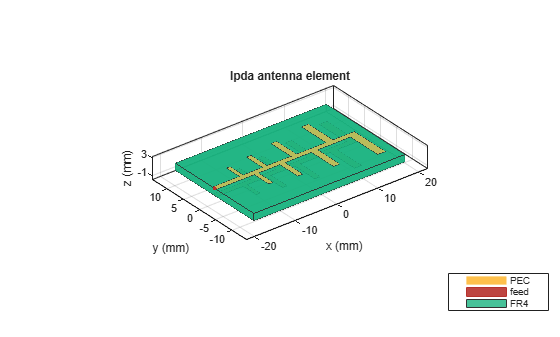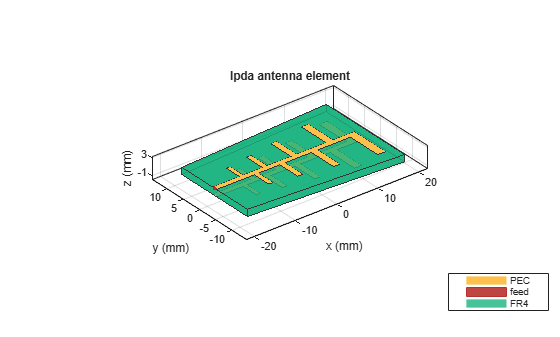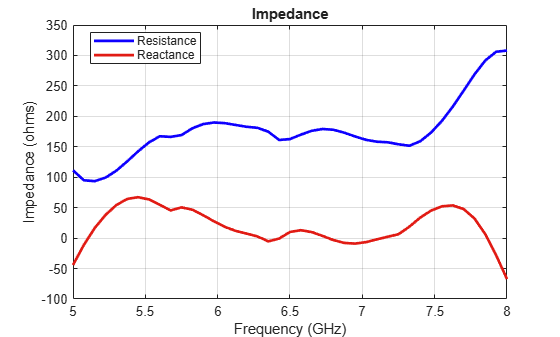# lpda

Create printed log-periodic dipole array antenna

## Description

Use the `lpda` object to create a printed log-periodic dipole array antenna. The default antenna is centered at the origin and uses an FR4 substrate. This antenna is widely used in communication and radar due to advantages such as wideband, high gain, and high directivity.## Creation

### Syntax

``lpdipole = lpda``
``lpdipole = lpda(Name,Value)``

### Description

example

````lpdipole = lpda` creates a printed log-periodic dipole array antenna using default property values.```

example

````lpdipole = lpda(Name,Value)` sets properties using one or more name-value pairs. For example, ```lpdipole = lpda('BoardLength',0.2)``` creates a printed log-periodic dipole array with a board length of 0.2 m. ```

Note

Properties which are not specified retain their default values.

## Properties

expand all

Printed circuit board (PCB) length along x-axis, specified as a scalar in meters.

Example: `'BoardLength',0.2`

Example: `lpdipole.BoardLength = 0.2`

Data Types: `double`

PCB width along y-axis, specified in meters. Width of the PCB in meter. If the value is a scalar, a rectangular board is created and if the value is a vector with 2 elements, a trapezoidal board is created. The first element represents width of the board at the shortest end of the dipole and the second element represents width at the longest end of the dipole.

Example: `'BoardWidth',[0.06 0.06]`

Example: ```lpdipole.BoardWidth = [10e-3 12e-3]```

Data Types: `double`

PCB height along z-axis, specified as a scalar in meters.

Example: `'Height',0.0018`

Example: `lpdipole.Height = 0.0018`

Data Types: `double`

Width of the parallel strip, specified as a scalar in meters.

Example: `'StripLineWidth',0.0014`

Example: `lpdipole.StripLineWidth = 0.0014`

Data Types: `double`

The distance from the feed point to the smallest dipole , specified as a scalar in meters.

Example: `'FeedLength',0.0055`

Example: `lpdipole.FeedLength = 0.0055`

Data Types: `double`

Lengths of individual dipole arms, specified as a vector with each element unit in meters.

Example: ```'ArmLength',[0.0050 0.0055 0.0060 0.0066 0.0072 0.0079 0.0086 0.0095]```

Example: ```lpdipole.ArmLength = [0.0050 0.0055 0.0060 0.0066 0.0072 0.0079 0.0086 0.0095]```

Data Types: `double`

Widths of individual dipole arms, specified as a vector with each element unit in meters.

Example: ```'ArmWidth',[9.8000e-04 10.8000e-04 0.0021 0.0022 0.0023 0.0025 0.0027 0.0029]```

Example: ```lpdipole.ArmWidth = [9.8000e-04 10.8000e-04 0.0021 0.0022 0.0023 0.0025 0.0027 0.0029]```

Data Types: `double`

Spacing between individual dipole arms, specified as a vector with each element unit in meters.

Example: ```'ArmSpacing',[0.0037 0.0040 0.0043 0.0047 0.0051 0.0056 0.0061]```

Example: ```lpdipole.ArmSpacing = [0.0037 0.0040 0.0043 0.0047 0.0051 0.0056 0.0061]```

Data Types: `double`

Type of dielectric material used as a substrate, specified as an dielectric object. For more information, see `dielectric`. For more information on dielectric substrate meshing, see Meshing.

Note

The substrate dimensions must be equal to the groundplane dimensions.

Example: ```d = dielectric('Teflon'); 'Substrate',d```

Example: ```d = dielectric('Teflon'); lpdipole.Substrate = d```

Type of the metal used as a conductor, specified as a metal material object. You can choose any metal from the `MetalCatalog` or specify a metal of your choice. For more information, see `metal`. For more information on metal conductor meshing, see Meshing.

Example: ```m = metal('Copper'); 'Conductor',m```

Example: ```m = metal('Copper'); ant.Conductor = m```

Lumped elements added to the antenna feed, specified as a lumped element object. You can add a load anywhere on the surface of the antenna. By default, the load is at the feed. For more information, see `lumpedElement`.

Example: `'Load',lumpedelement`, where `lumpedelement` is the object for the load created using `lumpedElement`.

Example: ```lpda.Load = lumpedElement('Impedance',75)```

Tilt angle of the antenna in degrees, specified as a scalar or vector. For more information, see Rotate Antennas and Arrays.

Example: `90`

Example: `Tilt=[90 90]`,`TiltAxis=[0 1 0;0 1 1]` tilts the antenna at 90 degrees about the two axes defined by the vectors.

Data Types: `double`

Tilt axis of the antenna, specified as one of these values:

• Three-element vector of Cartesian coordinates in meters. In this case, each coordinate in the vector starts at the origin and lies along the specified points on the x-, y-, and z-axes.

• Two points in space, specified as a 2-by-3 matrix corresponding to two three-element vectors of Cartesian coordinates. In this case, the antenna rotates around the line joining the two points.

• `"x"`, `"y"`, or `"z"` to describe a rotation about the x-, y-, or z-axis, respectively.

Example: `[0 1 0]`

Example: `[0 0 0;0 1 0]`

Example: `"Z"`

Data Types: `double` | `string`

## Object Functions

 `show` Display antenna, array structures, shapes, or platform `axialRatio` Axial ratio of antenna `beamwidth` Beamwidth of antenna `charge` Charge distribution on antenna or array surface `current` Current distribution on antenna or array surface `efficiency` Radiation efficiency of antenna `EHfields` Electric and magnetic fields of antennas or embedded electric and magnetic fields of antenna element in arrays `impedance` Input impedance of antenna or scan impedance of array `mesh` Mesh properties of metal, dielectric antenna, or array structure `meshconfig` Change meshing mode of antenna, array, custom antenna, custom array, or custom geometry `optimize` Optimize antenna or array using SADEA optimizer `pattern` Plot radiation pattern and phase of antenna or array or embedded pattern of antenna element in array `patternAzimuth` Azimuth plane radiation pattern of antenna or array `patternElevation` Elevation plane radiation pattern of antenna or array `rcs` Calculate and plot radar cross section (RCS) of platform, antenna, or array `returnLoss` Return loss of antenna or scan return loss of array `sparameters` Calculate S-parameters for antennas and antenna arrays `vswr` Voltage standing wave ratio (VSWR) of antenna or array element

## Examples

collapse all

Create and view a printed log-periodic dipole array antenna.

`lpdipole = lpda`
```lpdipole = lpda with properties: BoardLength: 0.0366 BoardWidth: 0.0244 Height: 0.0016 StripLineWidth: 0.0012 FeedLength: 0.0065 ArmLength: [0.0040 0.0045 0.0050 0.0056 0.0062 0.0069 0.0076 0.0085] ArmWidth: [8.8000e-04 9.8000e-04 0.0011 0.0012 0.0013 0.0015 0.0017 0.0019] ArmSpacing: [0.0027 0.0030 0.0033 0.0037 0.0041 0.0046 0.0051] Substrate: [1x1 dielectric] Conductor: [1x1 metal] Tilt: 0 TiltAxis: [1 0 0] Load: [1x1 lumpedElement] ```
`show(lpdipole)`Create a tapered LPDA object and plot impedance over a frequency of 5 - 8GHz. This example also shows how to plot the 3-D radiation pattern of the antenna.

```lpdipole = lpda('BoardWidth',[20.37e-3 24.37e-3]); show(lpdipole)```Plot Impedance over the specified frequency range.

```freq = linspace(5e9, 8e9, 41); figure; impedance(lpdipole,freq)```

Plot the 3-D radiation pattern at 5.8 GHz.

`pattern(lpdipole,5.8e9)`## Version History

Introduced in R2018a